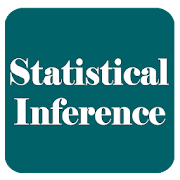# statistical inferenceThis app is educational app for students,, this app provide basic notes for students.
Statistical inference is very important course for BBA and another students.

Statistical inference is the process of using data analysis to deduce properties of an underlying probability distribution. Inferential statistical analysis infers properties of a population, for example by testing hypotheses and deriving estimates.
Important topics of statistical inference:
Statistical Inference
Population (macroscopic phenomenon) and Sample
Population parameter
Static
Estimator
Comparison of unbiased estimators
Inference
Confidence Interval
Hypothesis testing
Factors Increasing Type II Error
Hypothesis testing steps
Example normal body temperature